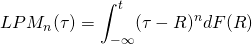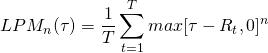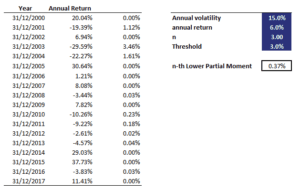# Lower Partial Moment (LPM)

A Lower Partial Moment (LPM) is a set of moments that is used to estimate downside risk in finance. In fact, there is not just one lower partial moment. Instead, we actually talk about the n-th lower partial moment, where n can be 1, 2, 3,… . Lower partial moments were first introduced by Harlow in 1991. Lower partial moments are often calculated to construct measures of downside risk and are needed to determine the Sortino ratio, the Omega ratio, and the Kappa ratio.

On this page, we discuss the lower partial moment definition, explain how to estimate the n-th lower partial moment, and finally provide an LPM calculator in Excel.

## Lower Partial Moment formula

The n-th LPM is defined as the following integralWhile this definition may look quite difficult to understand, its interpretation is actually quite straightforward. In particular, the LPM formula above models the moments of asset returns that fall below a certain minimum acceptable level of return tau. Thus, we calculate the moments of the returns that are below our minimum return. Similarly, we can also calculate the moments above the threshold tau. In that case, we refer to the moments as higher partial moments.

## Lower Partial Moment calculation

The n-th lower partial moment can be estimated using the following formulawhere T is the sample size and Rt is the t-th return. Thus, we only require return data to be able to calculate the lower partial moment of the data. The above estimation approach is a discrete calculation of the LPM. This is, however, not the only way we can estimate LPMs. An alternative way is to assume a certain (continuous) distribution and then calculate the integral directly. Here, we will continue to use the discrete approach, since the distribution of the returns is generally not known.

## Lower Partial Moment Excel

Now that we discussed the main idea behind LPMs as well as an easy way to estimate them in discrete time using the above formula, let’s put the formula to work. To get some intuition on using the LPM, we implemented the LPM in an Excel spreadsheet, which is available for download at the bottom of this page.

The following figure illustrates the necessary calculations## Summary

We discussed Lower Partial Moments (LPM), a method to quantify the moments of returns that fall short of a certain threshold. This method is becoming increasingly popular as downside risk is an important consideration. We show that investors can easily calculate LPMs using Excel. LPMs are used to calculate a number of risk-adjusted performance measures, such as the Sortino and the Omega ratio.

### LPM calculator

Want to have an implementation in Excel? Download the Excel file: Lower Partial Moment